# NCERT Solutions for Class 8 Maths Chapter 11 Mensuration (EX 11.1) Exercise 11.1

## NCERT Solutions for Class 8 Maths Chapter 11 Mensuration (EX 11.1) Exercise 11.1

Free PDF download of NCERT Solutions for Class 8 Maths Chapter 11 Exercise 11.1 (EX 11.1) and all chapter exercises at one place prepared by expert teacher as per NCERT (CBSE) books guidelines. Class 8 Maths Chapter 11 Mensuration Exercise 11.1 Questions with Solutions to help you to revise complete Syllabus and Score More marks. Register and get all exercise solutions in your emails.

Vedantu is a platform that provides free NCERT Solution and other study materials for students. Science Students who are looking for NCERT Solutions for Class 8 Science will also find the Solutions curated by our Master Teachers really Helpful.

### Important Formulas for NCERT Solutions Class 8 Maths Ch 11 Exercise 11.1

The sums given in NCERT Class 8 Maths Exercise 11.1 are to be solved using the formulas of areas and perimeters of shapes. It gives a brief overview of what we have learned so far in mensuration. Some of the common shapes like squares, rectangles, triangles, parallelograms, or circles are discussed in this section.

Here we have a list of formulas that you must remember to solve the sums given in NCERT Exercise 11.1 Mensuration Class 8.

 Sl.Nos. Shapes Formulas 1 Square Area = Side × SidePerimeter = 4 × Side 2 Rectangle Area = Length × BreadthPerimeter = 2 (Length + Breadth) 3 Triangle Area = 12 × Base × HeightPerimeter = Sum of all three sides 4 Circle Area = π × (radius)2Permitere = 2π × Radius 5 Parallelogram Area = Base × Perpendicular distance between the basesPerimeter = Sum of all four sides
Do you need help with your Homework? Are you preparing for Exams?
Study without Internet (Offline)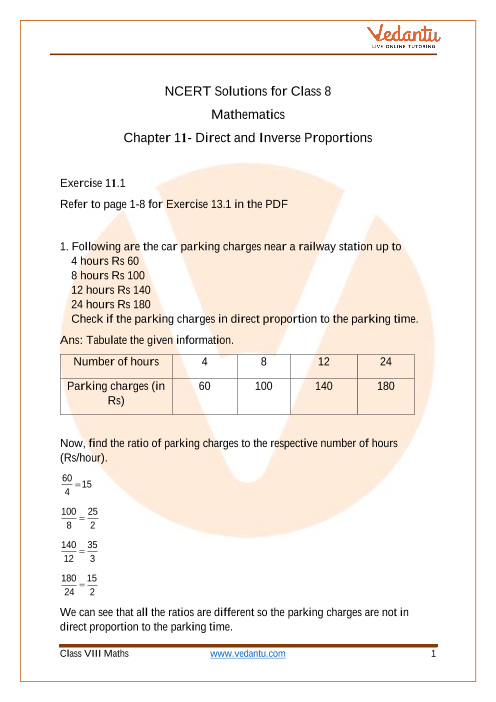Book your Free Demo session
Get a flavour of LIVE classes here at Vedantu## Access NCERT Solutions For Class 8 Maths Chapter 11 – Mensuration

Exercise (11.1)

1. A square and a rectangular filled with measurements as given in the figure have the same perimeter. Which field has a larger area?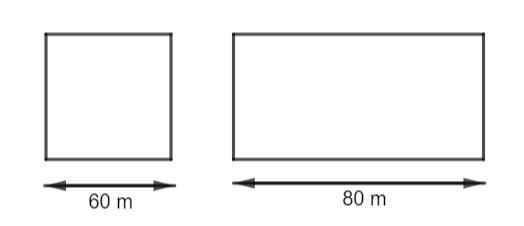Ans: Given that,

Side of the square $=60\text{ m}$

Length of the rectangle $=80\text{ m}$

Both the square and rectangle have same perimeter

To find,

Which field has larger area

Firstly to find the breadth of the rectangle

We know that,

Perimeter of the square = Perimeter of the rectangle

$4\left( \text{side of the square} \right)=2\left( length\times breadth \right)$

$4\left( 60 \right)=2l+2b$

$240=2\left( 80 \right)+2\left( b \right)$

$2b=240-160$

$2b=80$

$b=40\text{ m}$

Now area of the square$={{\left( side \right)}^{2}}$

$={{\left( 60 \right)}^{2}}$

$=3600\text{ }{{\text{m}}^{2}}$

Area of the rectangle $=length\times breadth$

$=80\times 40$

$=3200\text{ }{{\text{m}}^{2}}$

Area of the square > Area of the rectangle

$\therefore$ The area of the square has a comparatively larger area than the area of the rectangle.

2. Mrs. Kaushik has a square plot with the measurement as shown in the following figure. She wants to construct a house in the middle of the plot. A garden is developed around the house. Find the total cost of developing a garden around the house at the rate of $Rs.55$ per ${{m}^{2}}$.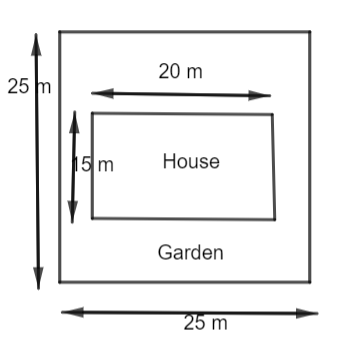Ans: Given that,

Side of the square plot $=25\text{ m}$

Length of the rectangular house $=20\text{ m}$

Breadth of the rectangular house $=15\text{ m}$

Cost of developing garden per ${{m}^{2}}=Rs.55$

To find,

The total cost of developing a garden around the house

Area of the square $={{\left( side \right)}^{2}}$

$={{\left( 25 \right)}^{2}}$

$=625\text{ }{{\text{m}}^{2}}$

Area of the rectangular house $=length\times breadth$

$=20\times 15$

$=300\text{ }{{\text{m}}^{2}}$

From the diagram, it can be noted that,

Area of the garden = Area of the square plot – Area of the rectangular house

$=625-300$

$=325\text{ }{{\text{m}}^{2}}$

Cost of developing garden per ${{m}^{2}}=Rs.55$

Cost of developing $325\text{ }{{\text{m}}^{2}}$ garden $=325\times 55$

$=Rs.17,875$

$\therefore$ The total cost for developing garden around the house will be $Rs.17,875$

3. The shape of a garden is rectangular in the middle and semi-circular at the ends as shown in the diagram. Find the area and the perimeter of the garden Length of rectangle is $20-\left( 3.5+3.5 \right)\text{ meters}$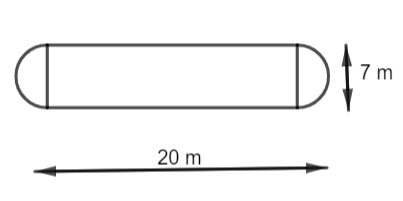Ans: Given that,

Total length of the garden $=20\text{ m}$

Length of the rectangular part $=20-\left( 3.5+3.5 \right)$

$=20-7$

$=13\text{ m}$

Breadth of the rectangular part $=7\text{ m}$

Diameter of semi-circular part$=7\text{ m}$

Radius of semi-circular part $=\dfrac{7}{2}$

$=3.5\text{ m}$

To find,

The perimeter of the garden and the area of the garden

Finding the perimeter of the garden

Perimeter of the garden = Perimeter of rectangular part + Perimeter of two semi-circular part

Perimeter of rectangular part $=2\left( l+b \right)$

$=2\left( 13+7 \right)$

$=2\left( 20 \right)$

$=40\text{ m}$

Perimeter of two semi-circular part $=2\left( \dfrac{2\pi r}{2} \right)$

$=2\pi r$

$=2\times \dfrac{22}{7}\times 3.5$

$=22\text{ m}$

Perimeter of the garden $=40+22$

$=62\text{ m}$

Area of the garden = Area of the rectangular part + Area of the two semi-circular part

Area of rectangular part $=l\times b$

$=13\times 7$

$=91\text{ }{{\text{m}}^{2}}$

Area of two semi-circular part $=2\left( \dfrac{\pi {{r}^{2}}}{2} \right)$

$=\pi {{r}^{2}}$

$=\dfrac{22}{7}\times 3.5\times 3.5$

$=38.5\text{ }{{\text{m}}^{2}}$

Area of the garden $=91+38.5$

$=129.5\text{ }{{\text{m}}^{2}}$

$\therefore$ The perimeter of the garden is $62\text{ m}$ and the area of the garden is $129.5\text{ }{{\text{m}}^{2}}$.

4. A flooring tile has the shape of a parallelogram whose base is $24\text{ cm}$ and the corresponding height is $10\text{ cm}$. How many such tiles are required to cover a floor of area $1080\text{ }{{\text{m}}^{2}}$?(If required you can split the tiles in whatever way you want to fill up the corners).

Ans: Given that,

Length of the parallelogram tile$=24\text{ cm}$

Breadth of the parallelogram tile$=10\text{ cm}$

Area of the floor with tiles $=1080\text{ }{{\text{m}}^{2}}$

Area of the a single parallelogram tile$= length\times breadth$

$=24\times 10$

$=240\text{ c}{{\text{m}}^{2}}$

Number of tiles required $=\dfrac{\text{Total area of the floor}}{Area\text{ of each tile}}$

We know that,

$1\text{ m}=100\text{ cm}$

$1\text{ }{{\text{m}}^{2}}=10000\text{ c}{{\text{m}}^{2}}$

$1080\text{ }{{\text{m}}^{2}}=1080\times 10000\text{ c}{{\text{m}}^{2}}$

$n=\dfrac{1080\times 10000}{240}$

$=45,000$ tiles

$\therefore 45,000$ tiles are required to make a floor.

5. An ant is moving around a few food pieces of different shapes scattered on the floor. For which food piece would the ant have to take a longer round? Remember, the circumference of a circle can be obtained by using the expression $c=2\pi r$, where $r$ is the radius of the circle.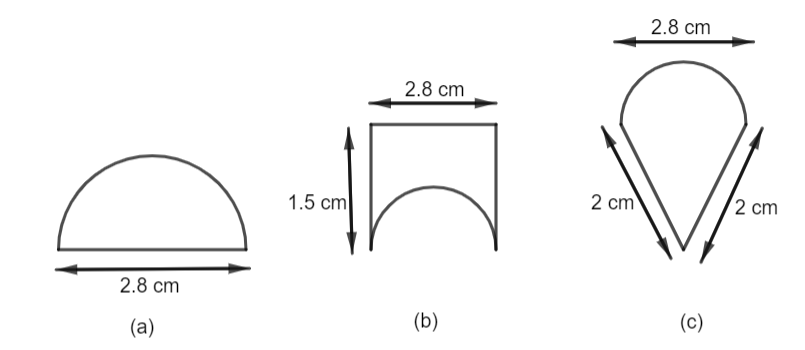Ans:

(a) Given that,

Diameter of the semicircle $=2.8\text{ cm}$

Radius of the semi-circle$=\dfrac{2.8}{2}$

$=1.4\text{ cm}$

Circumference of the semi-circle $=\dfrac{2\pi r}{2}$

$=\dfrac{22}{7}\times 1.4$

$=4.4\text{ cm}$

Total distance around the food piece (a) $\text{= Diameter+Perimeter of the semi-circle}$$= 2.8+4.4$

$=7.2\text{ cm}$

Total distance covered by ant in the food piece (a) is $7.2\text{ cm}$

(b) Given that,

Length of the given figure $=2.8\text{ cm}$

Breadth of the given figure $=1.5\text{ cm}$

Radius of semi-circular part $=\dfrac{2.8}{2}$

$=1.4\text{ cm}$

Perimeter of the semicircular part $=\dfrac{2\pi r}{2}$

$=\dfrac{22}{7}\times 1.4$

$=4.4\text{ cm}$

$=10.2\text{ cm}$

Total distance covered by ant in food piece (b) is $10.2\text{ cm}$

(c) Given that,

Two sides of the triangle are $2\text{ cm, 2 cm}$

Diameter of the semi-circular part $=2.8\text{ cm}$

Radius of the semi-circular part $=\dfrac{2.8}{2}$

$=1.4\text{ cm}$

Circumference of semi-circular part $=\dfrac{2\pi r}{2}$

$=\dfrac{22}{7}\times 1.4$

$=4.4\text{ cm}$

Total distance covered by ant in food piece (c)$=2+2+4.4$

$=8.4\text{ cm}$

$\therefore$ For the food piece (b), the ant have to take a longer time since the perimeter is comparatively larger for food piece (b)

## NCERT Solutions for Class 8 Maths Chapter 11 Mensuration (Ex 11.1) Exercise 11.1

Opting for the NCERT solutions for Ex 11.1 Class 8 Maths is considered as the best option for the CBSE students when it comes to exam preparation. This chapter consists of many exercises. Out of which we have provided the Exercise 11.1 Class 8 Maths NCERT solutions on this page in PDF format. You can download this solution as per your convenience or you can study it directly from our website/ app online.

Vedantu in-house subject matter experts have solved the problems/ questions from the exercise with the utmost care and by following all the guidelines by CBSE. Class 8 students who are thorough with all the concepts from the Subject Maths textbook and quite well-versed with all the problems from the exercises given in it, then any student can easily score the highest possible marks in the final exam. With the help of this Class 8 Maths Chapter 11 Exercise 11.1 solutions, students can easily understand the pattern of questions that can be asked in the exam from this chapter and also learn the marks weightage of the chapter. So that they can prepare themselves accordingly for the final exam.

Besides these NCERT solutions for Class 8 Maths Chapter 11 Exercise 11.1, there are plenty of exercises in this chapter which contain innumerable questions as well. All these questions are solved/answered by our in-house subject experts as mentioned earlier. Hence all of these are bound to be of superior quality and anyone can refer to these during the time of exam preparation. In order to score the best possible marks in the class, it is really important to understand all the concepts of the textbooks and solve the problems from the exercises given next to it.

Do not delay any more. Download the NCERT solutions for Class 8 Maths Chapter 11 Exercise 11.1 from Vedantu website now for better exam preparation. If you have the Vedantu app in your phone, you can download the same through the app as well. The best part of these solutions is these can be accessed both online and offline as well.

### NCERT Solutions Class 8 Maths Chapter 11 Other Exercises

 Chapter 11 Mensuration All Exercises in PDF Format Exercise 11.2 11 Questions and Solutions Exercise 11.3 10 Questions and Solutions Exercise 11.4 8 Questions and Solutions

### Chapter wise NCERT Solutions for Class 8 Maths

1. What does Class 8 Maths Chapter 11 of NCERT book depict?

Class 8 Maths Chapter 11 titled Mensuration deals with geometric figures and their parameters like length, volume, shape, surface area, lateral surface area, etc. Here, the questions/problems related to mensuration are explained thoroughly.

This chapter contains all the important mensuration formulas and properties of different geometric shapes and figures. The chapter also contains various problems related to perimeter and quadrilaterals which are solved by Vedantu’s top-notch subject matter experts.

2. How many questions are there in Class 8 Maths Chapter 11 Exercise 11.1 of NCERT textbook?

Class 8 Maths Chapter 11 Exercise 11.1 of NCERT textbook consists of five long answer type questions. Solutions to those problems have also been provided by Vedantu, India’s leading ed-tech portal. These solutions are created by the top-notch subject matter experts from the relevant industry.

3. What are the topics/ subtopics covered under the Class 8 Maths Chapter 11?

The topics/ subtopics covered under the Class 8 Maths Chapter 11 are as follows:

• 11.1 - Introduction

• 11.2 - Let us Recall

• 11.3 - Area of Trapezium

• 11.4 - Area of a General Quadrilateral

• 11.5 - Area of a Polygon

• 11.6 - Solid Shapes

• 11.7 - Surface Area of Cube, Cuboid and Cylinder

• 11.8 - Volume of Cube, Cuboid and Cylinder

• 11.9 - Volume and Capacity

4. Is it beneficial to refer to the NCERT Solutions Class 8 Maths Chapter 11 Exercise 11.1?

NCERT Solutions for Class 8 Maths Chapter 11 titled Mensuration contains all the answers for all the questions asked in the textbook. All the solutions are prepared by Vedantu’s subject matter experts as per the latest CBSE syllabus and guidelines.

NCERT Solutions Class 8 Maths Chapter 11 Exercise 11.1 helps all the students to prepare for the exams as per the syllabus and revise accordingly prior to the exam. These solutions will help the students to practise and learn the question and answer patterns as well. NCERT Solutions provided by Vedantu are of the best quality and help to score the highest possible marks in the final exam.

5. How many exercises and examples are there in Chapter 11 of Class 8 Maths including Exercise 11.1?

Chapter 11 of Class 8 Maths is “Mensuration”. The number of questions included in the Chapter and Exercise 11.1 is:

• Exercise 11.1 –five questions

• Exercise 11.2 –11 questions

• Exercise 11.3 – 10 questions

• Exercise 11.4 – eight questions

There are a total of 13 examples in this chapter.

By solving these questions, students will be able to understand the chapter easily. As it Is a calculative chapter, students have to practice more to grasp the concepts.

6. Show some differences between capacity and volume.

(a) Capacity:

• The ability of an object to contain a substance that is either solid, gas or liquid is termed as capacity.

• The other definition is, it is the amount of the potential of a substance that an object is able to hold.

• The SI units of capacity are gallons, litres, etc.

• Capacity is found in hollow objects.

(b) Volume:

• The total amount of space covered by the object is known as volume.

• It is defined as the actual amount of an object that covers a definite space.

• The SI unit of volume is cubic metres or cubic centimetres.

• Volume is found in both solid and hollow objects.

7. Write down the surface areas of the cylinder, cuboid and cube.

(a) Cube – The surface area of the cube is 6 * a^2.

(b) Cuboid –2 (lb + bh + hl) is the total surface area.

Here l is the length, b is the width and h is the height.

(c) Cylinder –2πrh is the curved surface area.

The total surface area of the cylinder is 2πr(h + r)

Here, r denotes the radius of the base and h is the height of the cylinder.

8. How can you say that area and perimeter are different?

(a) Perimeter:

• The boundary or outline of the shape of an object is known as the perimeter.

• The perimeter is measured in linear units.

• The perimeter is the sum of the length of all the sides of the object.

• For example, the perimeter of a square of length 10cm is 40cm.

(b) Area:

• The total space occupied by the object or the shape is called the area.

• It is measured in square units.

• For example, the area of the park which is square-shaped, 20cm long is 400 sq. cm.

9. Why should students study Chapter 11 Exercise 11.1 of Class 8 Maths from Vedantu?

Due to the following benefits, students prefer Vedantu for studying Exercise 11.1 of Chapter 11 of Class 8 Maths:

• All the NCERT Solutions of Exercise 11.1 of Chapter 11 of Class 8 Maths are accessible to the students free of cost on the Vedantu website and the Vedantu app.

• The well-qualified teachers are responsible for creating the content.

• The solutions are available in PDF files.

• The “Let’s Chat” option allows students to share their doubts with the teachers.

• As there is the option of “Download PDF”, students can even study offline.SHARETWEETSHARESUBSCRIBE